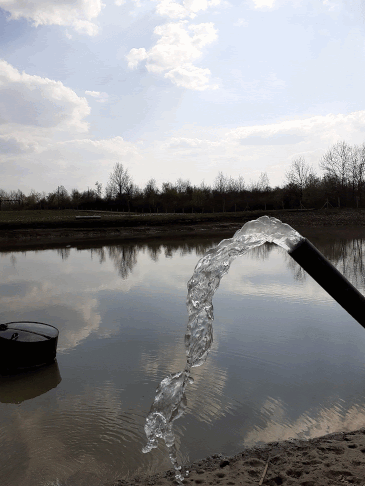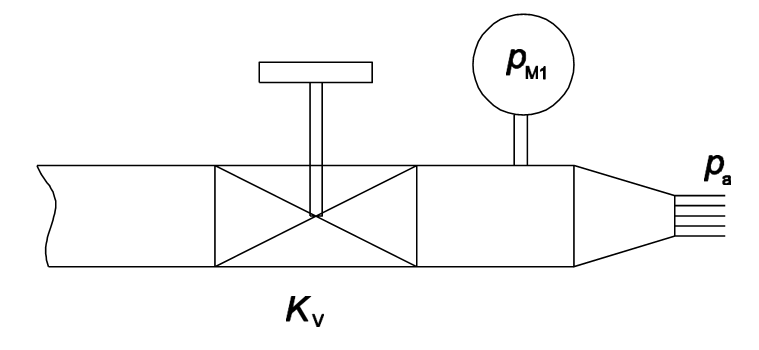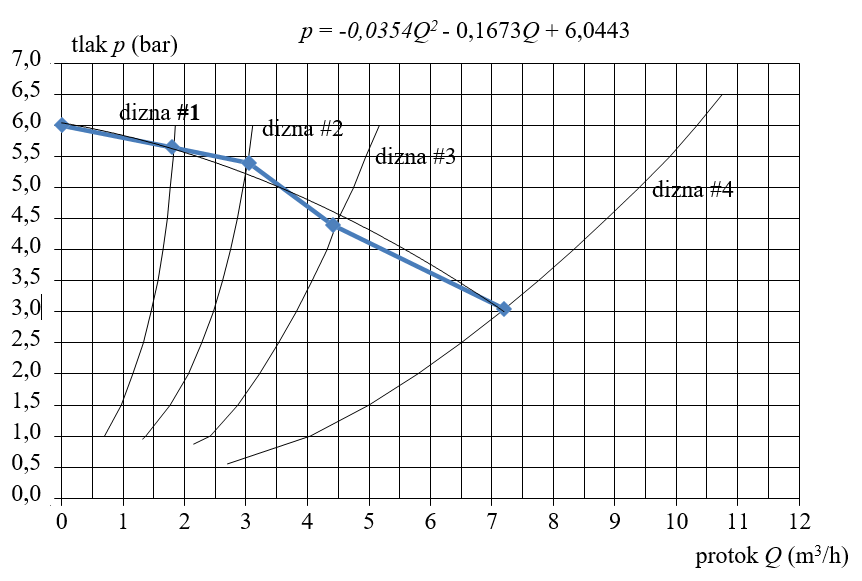## Blog posts & pages

View all results (0)The design of an antifrost irrigation or frost protection system begins with determining the characteristics of the water supply. The measurement is performed by determining the specific points u with the help of four calibrated nozzles p-Q diagram.Figure 1. Nozzles for measuring supply characteristics

Each nozzle has a specific flow curve depending on the pressure. When one nozzle is connected, the pressure for a certain flow according to its characteristic is read.Figure 2. Measurement scheme for determining supply characteristics

By connecting the four points thus obtained, one for each nozzle, the curve to be found is obtained This quadratic function curve is a characteristic of the water supply. It is then used to determine the flows depending on the pressures obtained. Such a curve describes our supply as a pump with the same characteristics. In case the supply unit is a pump, the supply characteristic is obtained from p-Q pump diagram by the same procedure of searching for a quadratic function.Figure 3. Pressure-dependent flow curve

* The results of the described test match because the results were checked for each nozzle. Flow meter used he measured the flow for each nozzle. The flows were compared with the catalog values of each nozzle for a given pressure. Deviations occur due to high pressure, so there is a loss in the insufficiently sealed nozzle connection to the system.